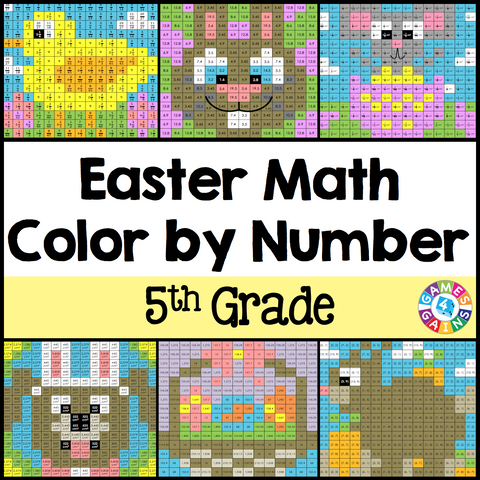## Easter Math Color-by-Number - 5th Grade

• \$480

Easter Math Color-by-Number set comes with 6 Easter Math color-by-number activities for reviewing 5th grade math skills. This Easter Math math set is perfect to use in centers, in small groups, or with the whole class!

Here are the skills covered by these Easter Math math color-by-number activities:

• Dividing decimals by decimals (up to hundredths)
• Multiplying fractions by fractions and whole numbers
• Determining the pattern to solve the missing number in a table
• Plotting points on the coordinate plane to form geometric shapes (types of triangles and quadrilaterals)
• Calculating area with fractional side lengths
• Calculating volume when 2 or more rectangular prisms are combined

Included with this Easter Math Math Color-by-Number set:
Instructions
6 color by number worksheets
6 student problem worksheets
6 teacher answer sheets

Want to learn more about what you'll get? Check out the preview to see a sample of the actual pages.